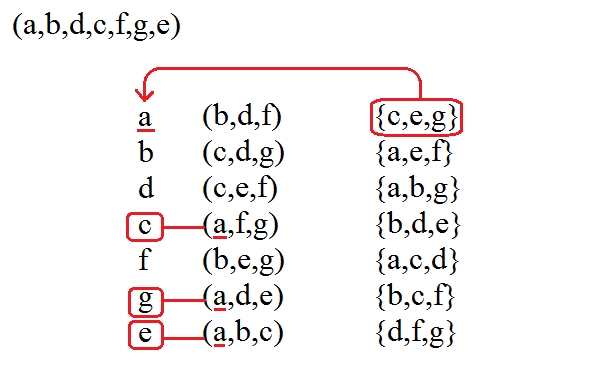None:
Polyps:
Strongs:RevelationHail And Fire Mingled With Blood The concepts of hail and fire are related to the "octal reversals" defined earlier. In starting with a seven cycle (a,b,d,c,f,g,e) and a static subgroup [b,d,f] we saw how the octal (additive) strucure was reversed in place upon the labels of singletons ad subgroups, i.e. in complete "reverse". However when we add the left handed column we se that the octal structure is actually in alignment with those of the singleton elements (although we actually permuted the subgroups downward.) So, a = 1 => [b,d,f] static with {c,e,g} b = 1 => [c,d,g] static with {a,e,f} d = 1 => [c,e,f] static with {a,b,g} (i) c = 1 => [a,f,g] static with {b,d,e} (ii) f = 1 => [b,e,g] static with {a,c,d} g = 1 => [a,d,e] static with {b,c,f} (ii) e = 1 => [a,b,c] static with {d,f,g} Now it seems strange that (i) + (ii) = (iii) in both singletons and the {...} (but not the [...]) As the additive structure is in alignment we have "hail" acting downward with the cycle in singletons and the {...} but it is in reversal acting "upward" with the [...]. to put it briefly fire "burns up" hail "falls down". Fire is often seen as the Holy Spirit in revelation. We note that if we fix unity as "a" then multiplying through by b = (a,b,d,c,f,g,e) actually maps b^-1 onto unity, so the last element "e" is now unity, and not "b" as we could surmise. Is it possible that this "hail and fire" are actually reversed? Have we been mistaken? We should be able to see from later references in the text whether this is so: but for now without loss of generality we carry on regardless. We have at least that the "right hand" is indeed hot either way! (it is never lukewarm). The "Blood" becomes apparent when K4 groups of subgroups in the left hand are examined with their intersection. They correspond in place in the alignment to those same elements in singletons that comprise the right handed subgroup corresponding to the intersection (of the left handed subgroups) itself. This is symmetrical with respect to both left and right hands and forms a "circulatory" style system between the left and right hands and their subgroups. It is this intersection property that we may place in a 1-1 correspondence to avoid a problem of infinite descent.We use the terms "fire column" and "hail column" to refer to this generation of [...] and {...} in the examination of the text. For now, simply understand the concept of octal reversal and alignment with the remaining hand. Continue To Next Page Return To Section Start Return To Previous Page'